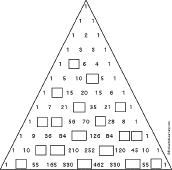EnchantedLearning.com is a user-supported site.
As a bonus, site members have access to a banner-ad-free version of the site, with print-friendly pages.

 You might also like: Polynomials Algebra: Solve the quadratic equations Worksheet #2 Printout Algebra: Multiplying Polynomials Worksheet #2 Printout Algebra: Quadratic Equations with No Linear Term Algebra Worksheets: Quadratic Equations with No Linear Term Today's featured page: Frogs and Toads

 Pascal's Triangle More MathGo to the answers

Pascal's triangle is a triangular pattern of numbers devised in 1653 by the French mathematician, physicist, and philosopher Blaise Pascal (June 19, 1623 - Aug. 19, 1662). The numbers in each row are derived by adding pairs of numbers in the row above it. The number one is tacked on the beginning and end of each row.

The first few rows of Pascal's triangle are shown below; fill in the rest of the triangle on the worksheet. Note that Pascal's triangle is infinite (it goes on forever).Pascal's Triangle Worksheet." The full-size printout is available only to site members.

## Enchanted Learning Search

 Search the Enchanted Learning website for: